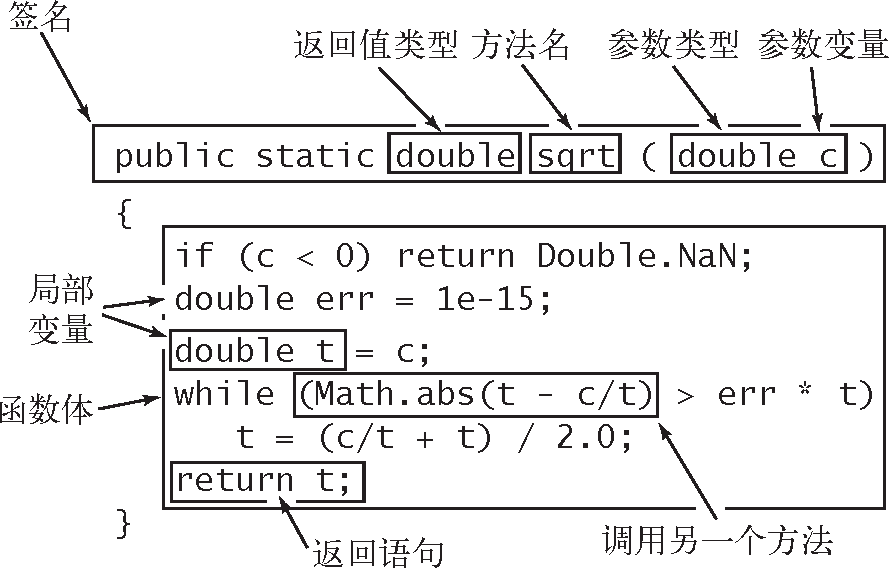# 算法（4th ed）(29)：基础——基础编程模型 3.6.1(静态方法：静态方法）public static int abs(int x){    if (x < 0) return -x;    else       return x;}

public static double abs(double x){    if (x < 0.0) return -x;    else         return x;}

public static boolean isPrime(int N){    if (N < 2) return false;    for (int i = 2; i*i <= N; i++)        if (N % i == 0) return false;    return true;}

public static double sqrt(double c){    if (c < 0) return Double.NaN;    double err = 1e-15;    double t = c;    while (Math.abs(t - c/t) > err * t)        t = (c/t + t) / 2.0;    return t;}

public static double hypotenuse(double a, double b){ return Math.sqrt(a*a + b*b); }

public static double H(int N){    double sum = 0.0;    for (int i = 1; i <= N; i++)        sum += 1.0 / i;    return sum;}